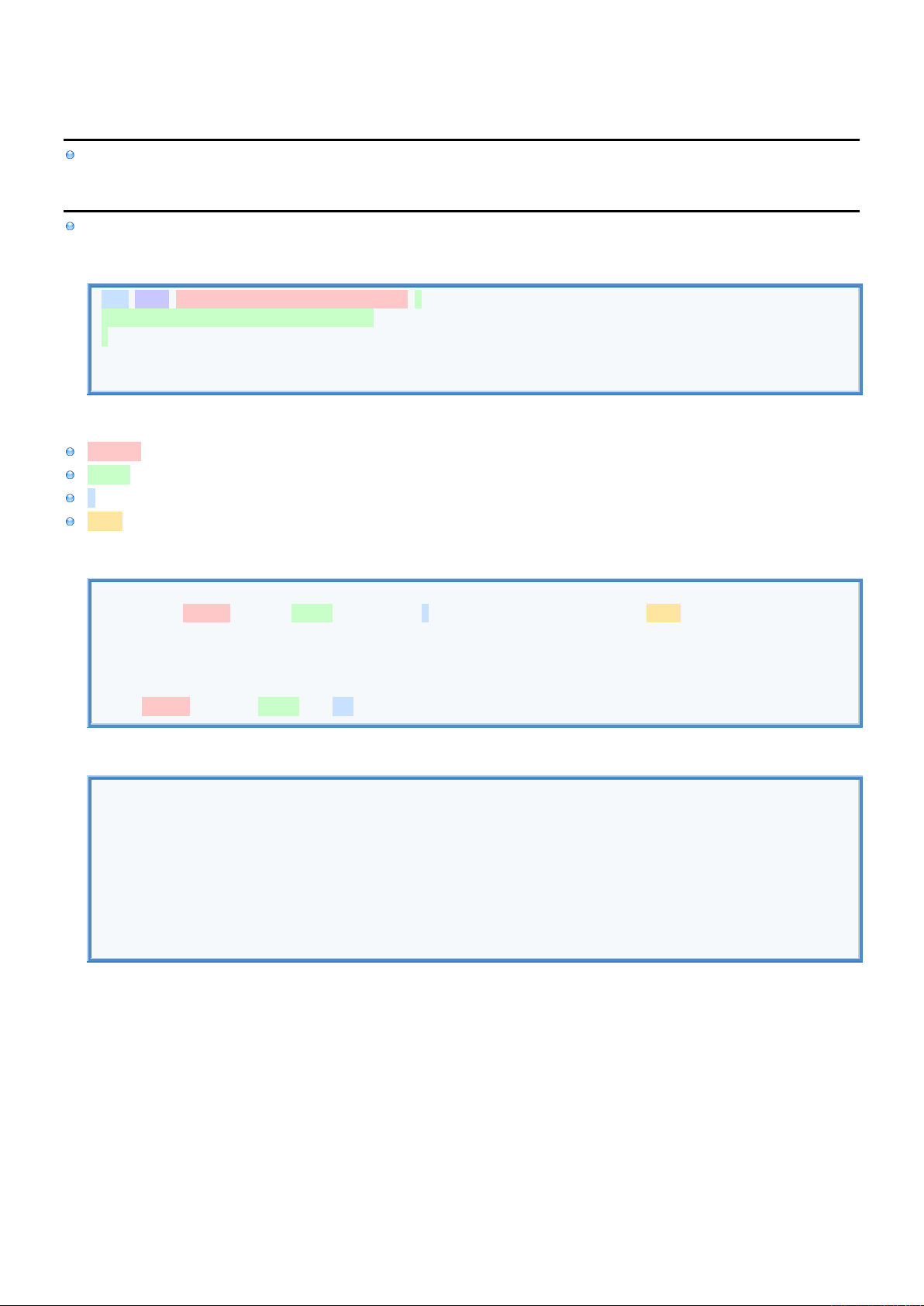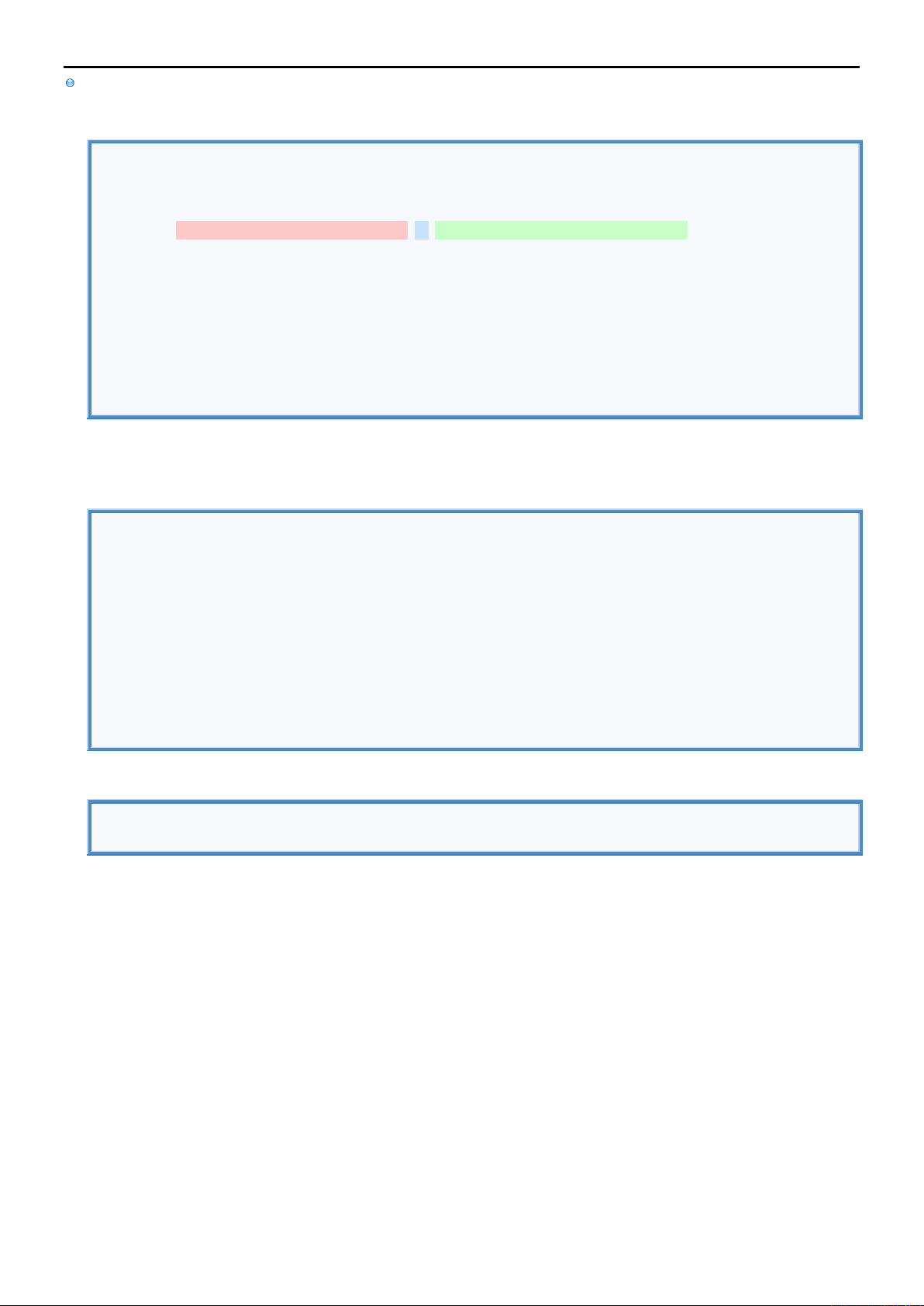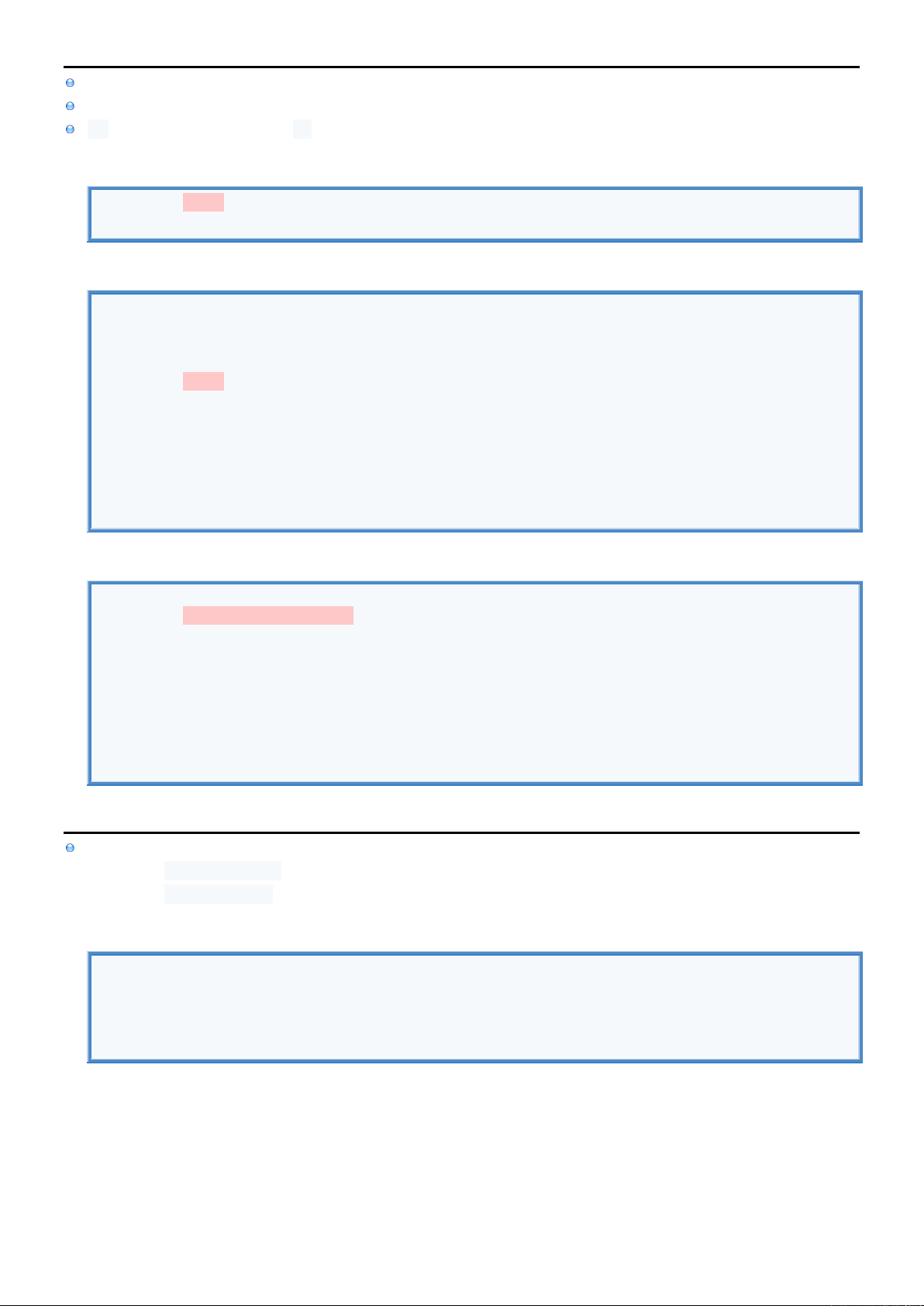3
3
.
.
5
5
F
F
u
u
n
n
c
c
t
t
i
i
o
o
n
n
s
s
I
I
n
n
f
f
o
o
This summary covers: Functions, Closures, Subscripts, Computed Property, Property Observers
F
F
u
u
n
n
c
c
t
t
i
i
o
o
n
n
s
s
Function can't have indexed parameters (like Closure can: \$0)
Basic Syntax
func greet (name:String, age:Int) -> (String) { //DECLARE FUNCTION.
return("\(name) is \(age) years old.") //IMPLEMENT FUNCTION.
}
var result = greet(name: "John", age:20) //CALL FUNCTION.
print(result) //DISPLAY RESULT.
I
I
n
n
p
p
u
u
t
t
P
P
a
a
r
r
a
a
m
m
e
e
t
t
e
e
r
r
s
s
nameInt is required Internal name used inside the Function to reference Parameter.
ageExt is optional External Name which must be used during Function call (otherwise internal name is used)
_ means that Parameter can be called without name
= 180 is default value which is given to Parameter if Parameter is omitted during call
Input Parameters
//DECLARE FUNCTION.
func greet (nameInt:String, ageExt ageInt:Int, _ weightInt: Int, heightInt: Int = 180) -> () {
print("\(nameInt) is \(ageInt) years old and \(weightInt) kg heavy and \(heightInt) cm tall.")
}
//CALL FUNCTION.
greet(nameInt: "John", ageExt:20, 150)
Input-Output Parameters
//DECLARE FUNCTION.
func greet (name: inout String) -> () { name = "John" }
//CALL FUNCTION.
var person = "Unknown"
greet(name: &person) //Give reference instead of value.
//DISPLAY CHANGED NAME.
print(person)R
R
e
e
t
t
u
u
r
r
n
n
V
V
a
a
l
l
u
u
e
e
s
s
Return Data Type must be explicitly given
Return String
func greet () -> (String) { return("Hello") } //DECLARE FUNCTION.
var result = greet() //CALL FUNCTION.
print(result) //DISPLAY RETURN VALUE.
Return Tuple
//DECLARE FUNCTION.
func greet () -> (name: String, age: Int) { return("John", 20) } //Returns Tuple with Named Parameters
func greet1 () -> ( String, Int) { return("John", 20) } //Returns Tuple with Indexed Parameters
//CALL FUNCTION. STORE INTO TUPLE.
var result = greet()
print(result)
print(result.0)
print(result.name)
//CALL FUNCTION. STORE INTO VARIABLES.
var (nameRet, ageRet) = greet()
print(nameRet)
print(ageRet)
Return struct
//DECLARE STRUCT.
struct Person {
var name : String
var age : Int
}
//DECLARE FUNCTION.
func greet () -> (Person) {
var person = Person(name: "John", age: 20)
return(person)
}
//CALL FUNCTION.
var person = greet()
//DISPLAY RETURN VALUE.
print(person)C
C
l
l
o
o
s
s
u
u
r
r
e
e
s
s
Return Data Type can be implicit
Closure Syntax
//DECLARE VARIABLE THAT CAN HOLD CLOSURE DATA TYPE (OF SPECIFIED SIGNATURE).
var greet : (String, Int) -> (String) //For Closures that have 2 Parameters & return String
//DECLARE CLOSURE. ASSIGN CLOSURE TO VARIABLE.
greet = { (name:String, age:Int) -> (String) in return("\(name) is \(age) years old") }
greet = { (name , age ) -> (String) in return("\(name) is \(age) years old") }
greet = { (name , age ) in return("\(name) is \(age) years old") }
greet = { name , age in return("\(name) is \(age) years old") }
//CALL CLOSURE. STORE RETURN VALUE INTO VARIABLE.
var result = greet("John", 20) //Closure can't have named Parameters.
//DISPLAY RESULT.
print(result)
I
I
n
n
p
p
u
u
t
t
P
P
a
a
r
r
a
a
m
m
e
e
t
t
e
e
r
r
s
s
Input Parameters
//STORE CLOSURE INTO VARIABLE.
var greet : (String, Int) -> (String)
//DECLARE CLOSURE. ASSIGN CLOSURE TO VARIABLE.
greet = { name, age in return("\(name) is \(age) years old") } //Use names
greet = { return("\(\$0) is \(\$1) years old") } //Use indexes
//CALL CLOSURE. STORE RETURN VALUE INTO VARIABLE.
var result = greet("John", 20) //Closure can't have named Parameters.
//CALL CLOSURE.
print(result)
No Input Parameters & no return value
var greet : () -> () = { print("John is 20 years old") }
greet()R
R
e
e
t
t
u
u
r
r
n
n
V
V
a
a
l
l
u
u
e
e
s
s
Return Data Type can be implicit
Compared to Function declaration just add red parts.
Return String
let greet : () -> (String) = { return("Hello") } //STORE CLOSURE INTO VARIABLE. No input Parameters.
var result = greet() //CALL CLOSURE.
print(result) //DISPLAY RETURN VALUE.
Return Tuple
//DECLARE VARIABLES.
var greet : () -> (name: String, age: Int)
var greet1 : () -> ( String, Int)
//STORE CLOSURES.
greet = { () -> (String, Int) in return("John", 50) } //Explicit Return Data Type. Names would be ignored.
greet = { return("John", 50) } //Implicit Return Data Type. Taken from Variable.
//CALL CLOSURE. STORE INTO TUPLE.
var result = greet() //Store Tuple into another Tuple.
print(result)
print(result.0)
print(result.name) //Variable names are always used
//CALL CLOSURE. STORE INTO VARIABLES.
var (nameRet, ageRet) = greet() //Store Tuple values into Variables.
print(nameRet)
print(ageRet)
Return struct
//DECLARE STRUCT.
struct Person {
var name : String
var age : Int
}
//STORE CLOSURE INTO VARAIBLE.
let greet : () -> (Person) = {
var person = Person(name: "John", age: 20)
return(person)
}
//CALL CLOSURE.
var person = greet()
//DISPLAY RETURN VALUE.
print(person)S
S
u
u
b
b
s
s
c
c
r
r
i
i
p
p
t
t
s
s
You can think of Subscript as a Method that is referenced using square brackets like john["name"].
For each subscript you need to define Data Type
used for the index (Input Parameter)
that is returned (Return Value)
Access Properties through Subscript
//DECLARE CLASS.
class Employee {
//DECLARE FIELDS.
var name = "unknown"
var position = "unknown";
//DECLARE SUBSCRIPT.
subscript (index: String) -> (String) {
get {
switch (index) {
case "name" : return name
case "position" : return position
default : return "unknown index";
}
}
set { //set (myValue) {...}
switch (index) {
case "name" : name = newValue //newValue is default
case "position" : position = newValue
default : print("unknown index");
}
}
}
}
//CREATE OBJECT.
var employee = Employee()
//USE SUBSCRIPTS TO STORE DATA INTO FIELDS.
employee["name" ] = "John" //Calls set
employee["position"] = "Manager" //Calls set
//USE SUBSCRIPTS TO RETRIEVE DATA FROM FIELDS.
print(employee["name" ]) //Calls get
print(employee["position"]) //Calls getC
C
o
o
m
m
p
p
u
u
t
t
e
e
d
d
P
P
r
r
o
o
p
p
e
e
r
r
t
t
y
y
Computed Property uses getter and setter Methods to compute (store & retrieve) values from other Variables/Methods.
Even though they are just Methods you reference them as if they were Variables.
get can be omitted if there is no set.
Only implicit getter
var greet : String { "Hello John" } //Return can be ommited for single line
print(greet)
Explicit getter & setter
//DECLARE VARIABLES.
var name : String = "" //Normal Variable where value is acctualy stored
//DECLARE COMPUTED PROPERTY.
var greet : String {
get { return "Hello \(name)" } //Composes greeting
set { name = newValue } //Stores new name (newValue is default). set (myValue) {...}
}
//REFERENCE COMPUTED PROPERTY.
greet = "John" //Calls set which stores "John" into name Variable
print(greet) //Calls get which returns "Hello John" by using name Variable
Computed Property returns Closure
//DECLARE COMPUTED PROPERTY.
var greet : (String, Int) -> (String) { //Only has get
var closure : (String, Int) -> (String) = { name, age in return("\(name) is \(age) years old") }
return(closure)
}
//REFERENCE COMPUTED PROPERTY.
var closure : (String, Int) -> (String) = greet //Calls get which returns Closure.
var result = closure("John", 50) //Call Closure
print(result)
P
P
r
r
o
o
p
p
e
e
r
r
t
t
y
y
O
O
b
b
s
s
e
e
r
r
v
v
e
e
r
r
s
s
Property Observers are methods that can be added to any variable, constant or field
Method willSet (newValue) is called before the new value is stored
Method didSet (oldValue) is called after the new value is stored
Property Observer Syntax
var name : String = "John" {
willSet { print("Name will be changed from \(name) to \(newValue)") } //willSet (newValue) {...}
didSet { print("Name was changed from \(oldValue) to \(name)" ) } //didSet (oldValue) {...}
}
name = "Bill"